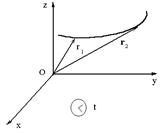CATEGORIES:

# Measurement

When you can measure what you are speaking about, and express it in numbers, you know something about it; but when you cannot express it in numbers, your knowledge is of a meager and unsatisfactory kind; it may be the beginning of knowledge, but you have scarcely, in your thoughts, advanced to the stage of Science, whatever the matter may be. Often in the history of science small but significant discrepancies between the theory and accurate measurements have led to the development of new and more general theories. Such advances in our understanding would not have occurred if scientists had been satisfied with only a qualitative explanation of the phenomena of nature.

The building blocks of physics are the physical quantities in terms of which the laws of physics are expressed. Among these are force, time, velocity, density, temperature, charge, magnetic susceptibility, and numerous others. When these terms are used, their meanings may be vague or may differ from their scientific meanings.

For the purposes of physics the basic quantities must be defined clearly and precisely. Physical quantities are often divided into fundamental quantities and derived quantities. Derived quantities are whose defining operations are based on other physical quantities. For example:. (1.1)

Fundamental quantities are not defined in terms of other physical quantities. Their operational definitions involve two steps: first, the choice of a standard, and second, the establishment of procedures for comparing the standard to the quantity to be measured so that a number and a unit are determined as the measure of that quantity.

In general, the measured value of a physical quantity depends on the reference frame of the observer making the measurement. Consider reference frames moving with uniform velocity with respect to each other and with respect to the fixed stars. Such reference frames are called inertial frame of reference. Experiment shows that all inertial reference frames are equivalent for the measurement of physical phenomena. Observers in different frames may obtain different numerical values for measured physical quantities, but the relationships between the measured quantities, that is, the laws of physics, will be the same for all observers.Figure 1.1

Three different systems of units are most commonly used in science and engineering. They are the meter-kilogram-second or MKS system, the Gaussian system, in which the fundamental mechanical units are the centimeter, the gram, and the second – CGS system, and the British engineering system (a foot-pound-second).

Date: 2015-01-12; view: 824

 <== previous page | next page ==> Lecture 18. The second law of thermodynamics ………………. …..…. 127 | Vectors and scalars
doclecture.net - lectures - 2014-2023 year. Copyright infringement or personal data (0.012 sec.)